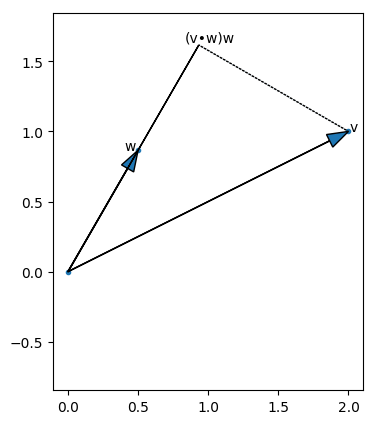# Linear algebra¶

Linear algebra is the branch of mathematics that deals with vector spaces.

```import re, math, random # regexes, math functions, random numbers
import matplotlib.pyplot as plt # pyplot
from collections import defaultdict, Counter
from functools import partial, reduce
```

## Vectors¶

Vectors are points in some finite-dimensional space.

```v = [1, 2]
w = [2, 1]
vectors = [v, w]
```
```def vector_add(v, w):
return [v_i + w_i for v_i, w_i in zip(v,w)]
```
```vector_add(v, w)
```
```[3, 3]
```
```def vector_subtract(v, w):
"""subtracts two vectors componentwise"""
return [v_i - w_i for v_i, w_i in zip(v,w)]

vector_subtract(v, w)
```
```[-1, 1]
```
```def vector_sum(vectors):
```
```vector_sum(vectors)
```
```[3, 3]
```
```def scalar_multiply(c, v):
# c is a number, v is a vector
return [c * v_i for v_i in v]
```
```scalar_multiply(2.5, v)
```
```[2.5, 5.0]
```
```def vector_mean(vectors):
"""compute the vector whose i-th element is the mean of the
i-th elements of the input vectors"""
n = len(vectors)
return scalar_multiply(1/n, vector_sum(vectors))
```
```vector_mean(vectors)
```
```[1.5, 1.5]
```
```def dot(v, w):
"""v_1 * w_1 + ... + v_n * w_n"""
return sum(v_i * w_i for v_i, w_i in zip(v, w))
```
```dot(v, w)
```
```4
```

The dot product measures how far the vector v extends in the w direction.

• For example, if w = [1, 0] then dot(v, w) is just the first component of v.

The dot product measures the length of the vector you’d get if you projected v onto w.

```def sum_of_squares(v):
"""v_1 * v_1 + ... + v_n * v_n"""
return dot(v, v)
```
```sum_of_squares(v)
```
```5
```
```def magnitude(v):
return math.sqrt(sum_of_squares(v))
```
```magnitude(v)
```
```2.23606797749979
```
```def squared_distance(v, w):
return sum_of_squares(vector_subtract(v, w))
```
```squared_distance(v, w)
```
```2
```
```def distance(v, w):
return math.sqrt(squared_distance(v, w))
```
```distance(v, w)
```
```1.4142135623730951
```

Using lists as vectors

• is great for exposition

• but terrible for performance.

• to use the NumPy library.

## Matrices¶

A matrix is a two-dimensional collection of numbers.

• We will represent matrices as lists of lists

• If A is a matrix, then A[i][j] is the element in the ith row and the jth column.

```A = [[1, 2, 3],
[4, 5, 6]]

B = [[1, 2],
[3, 4],
[5, 6]]
```
```def shape(A):
num_rows = len(A)
num_cols = len(A) if A else 0
return num_rows, num_cols
```
```shape(A)
```
```(2, 3)
```
```def get_row(A, i):
return A[i]
```
```get_row(A, 1)
```
```[4, 5, 6]
```
```def get_column(A, j):
return [A_i[j] for A_i in A]
```
```get_column(A, 2)
```
```[3, 6]
```
```def make_matrix(num_rows, num_cols, entry_fn):
"""returns a num_rows x num_cols matrix
whose (i,j)-th entry is entry_fn(i, j),
entry_fn is a function for generating matrix elements."""
return [[entry_fn(i, j)
for j in range(num_cols)]
for i in range(num_rows)]
```
```def entry_add(i, j):
"""a function for generating matrix elements. """
return i+j

```
```[[0, 1, 2, 3, 4],
[1, 2, 3, 4, 5],
[2, 3, 4, 5, 6],
[3, 4, 5, 6, 7],
[4, 5, 6, 7, 8]]
```
```def is_diagonal(i, j):
"""1's on the 'diagonal',
0's everywhere else"""
return 1 if i == j else 0

identity_matrix = make_matrix(5, 5, is_diagonal)
identity_matrix
```
```[[1, 0, 0, 0, 0],
[0, 1, 0, 0, 0],
[0, 0, 1, 0, 0],
[0, 0, 0, 1, 0],
[0, 0, 0, 0, 1]]
```

### Matrices will be important.¶

• using a matrix to represent a dataset

• using an n × k matrix to represent a linear function that maps k-dimensional vectors to n-dimensional vectors.

• using matrix to represent binary relationships.

``` friendships = [(0, 1),
(0, 2),
(1, 2),
(1, 3),
(2, 3),
(3, 4),
(4, 5),
(5, 6),
(5, 7),
(6, 8),
(7, 8),
(8, 9)]
```
```friendships = [[0, 1, 1, 0, 0, 0, 0, 0, 0, 0], # user 0
[1, 0, 1, 1, 0, 0, 0, 0, 0, 0], # user 1
[1, 1, 0, 1, 0, 0, 0, 0, 0, 0], # user 2
[0, 1, 1, 0, 1, 0, 0, 0, 0, 0], # user 3
[0, 0, 0, 1, 0, 1, 0, 0, 0, 0], # user 4
[0, 0, 0, 0, 1, 0, 1, 1, 0, 0], # user 5
[0, 0, 0, 0, 0, 1, 0, 0, 1, 0], # user 6
[0, 0, 0, 0, 0, 1, 0, 0, 1, 0], # user 7
[0, 0, 0, 0, 0, 0, 1, 1, 0, 1], # user 8
[0, 0, 0, 0, 0, 0, 0, 0, 1, 0]] # user 9
```
```friendships == 1 # True, 0 and 2 are friends
```
```True
```
```def matrix_add(A, B):
if shape(A) != shape(B):
raise ArithmeticError("cannot add matrices with different shapes")

num_rows, num_cols = shape(A)
def entry_fn(i, j): return A[i][j] + B[i][j]

return make_matrix(num_rows, num_cols, entry_fn)
```
```A = make_matrix(5, 5, is_diagonal)

```
```[[1, 1, 2, 3, 4],
[1, 3, 3, 4, 5],
[2, 3, 5, 5, 6],
[3, 4, 5, 7, 7],
[4, 5, 6, 7, 9]]
```
```v = [2, 1]
w = [math.sqrt(.25), math.sqrt(.75)]
c = dot(v, w)
vonw = scalar_multiply(c, w)
o = [0,0]
plt.figure(figsize=(4, 5), dpi = 100)

plt.arrow(0, 0, v, v,
plt.annotate("v", v, xytext=[v + 0.01, v])

plt.arrow(0 ,0, w, w,